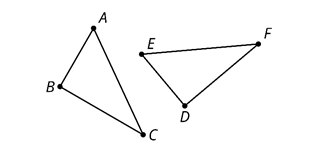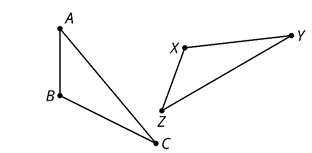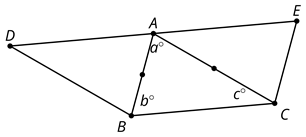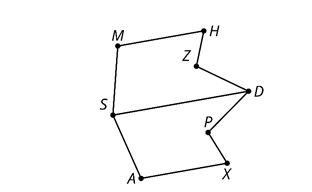# G.2.3 In Class Practice

Triangle ABC is congruent to triangle EDF. So, Kiran knows that there is a sequence of rigid motions that takes ABC to EDF.  Select all true statements after the transformations:Select all that apply
• A
• B
• C
• D
• E

The triangles are congruent. Which sequence of rigid motions will take triangle XYZ onto triangle BCA?Select all that apply
• A
• B
• C
• D
Line DE is parallel to line BC.a. What is the measure of angle EAC? b. What is the measure of angle DAB?
Line SD is a line of symmetry for figure ASMHZDPX. Tyler says that ASDPX is congruent to SMDZH because sides AS and MS are corresponding.a. Why is Tyler's congruence statement incorrect? b. Write a correct congruence statement for the pentagons.